Intro to the Number Line Math Video for Kids - K, 1st, & 2nd Grades
1%
It was processed successfully!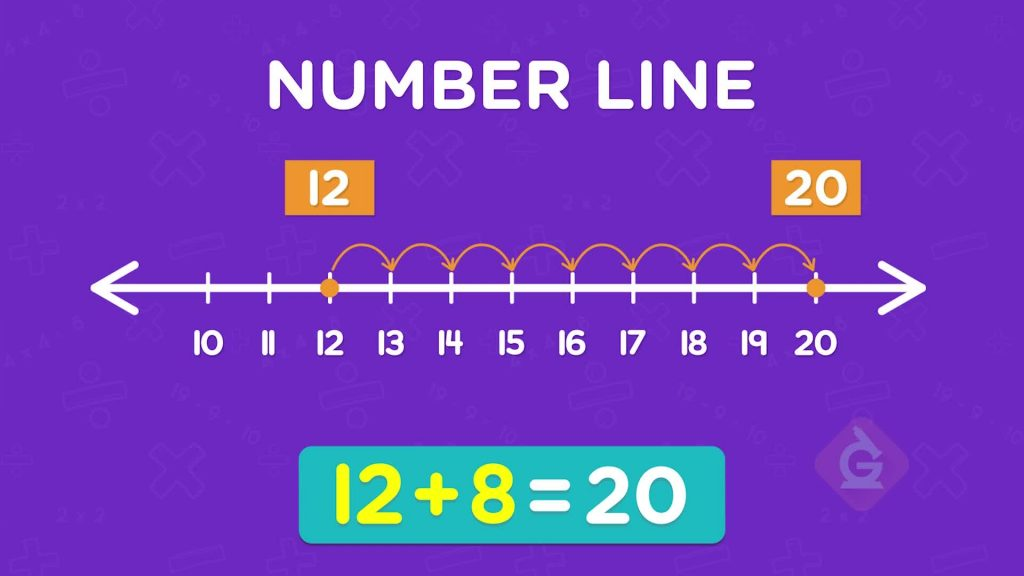WHAT IS A NUMBER LINE?

A number line is a line that shows numbers, in order. A number line can start at any number and end at any number. You can use number lines to count, add, and subtract.

To better understand number lines…

WHAT IS A NUMBER LINE?. A number line is a line that shows numbers, in order. A number line can start at any number and end at any number. You can use number lines to count, add, and subtract. To better understand number lines…

## LET’S BREAK IT DOWN!

### Compare numbers of jelly beans.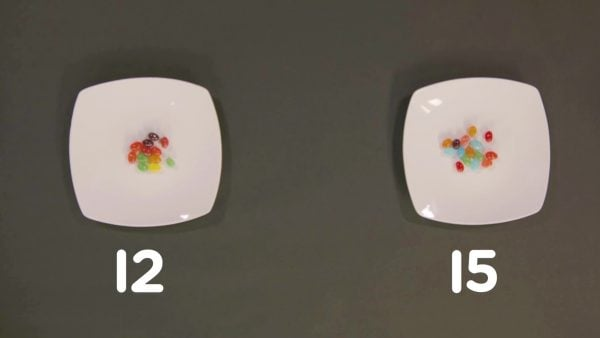You have 12 jelly beans. Your friend has 15 jelly beans. Who has more? You can compare numbers on a number line. Number lines have tick marks to mark numbers. Numbers increase from left to right. 15 is to the right of 12 on a number line. So, 15 is greater.

Compare numbers of jelly beans. You have 12 jelly beans. Your friend has 15 jelly beans. Who has more? You can compare numbers on a number line. Number lines have tick marks to mark numbers. Numbers increase from left to right. 15 is to the right of 12 on a number line. So, 15 is greater.

### Add numbers of parrot seeds.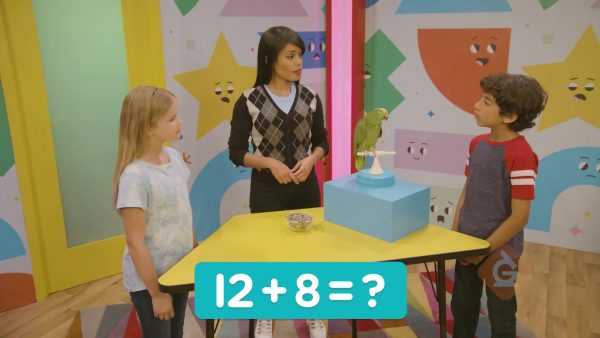You give your parrot 12 seeds in the morning and 8 seeds in the afternoon. You can use a number line to find the total number of seeds you give your parrot each day: 12 + 8. First, mark the 12 on the number line. Then jump 8 numbers to the right. This is like counting on 8 numbers. The last number you land on is 20. So, 12 + 8 = 20.

Add numbers of parrot seeds. You give your parrot 12 seeds in the morning and 8 seeds in the afternoon. You can use a number line to find the total number of seeds you give your parrot each day: 12 + 8. First, mark the 12 on the number line. Then jump 8 numbers to the right. This is like counting on 8 numbers. The last number you land on is 20. So, 12 + 8 = 20.

### Add 2-digit numbers on a number line.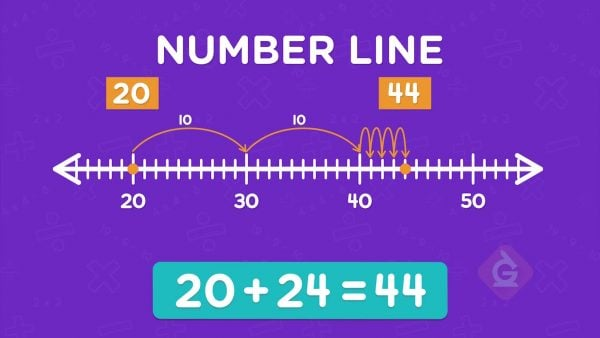You can add bigger numbers in parts by skip counting. Find 20 + 24. Start at 20 on the number line. One way to jump 24 is to jump 10 and 10 and 4. The last number you land on is 44. 20 + 24 = 44. You could make jumps of any size, as long as you jump 24 in all.

Add 2-digit numbers on a number line. You can add bigger numbers in parts by skip counting. Find 20 + 24. Start at 20 on the number line. One way to jump 24 is to jump 10 and 10 and 4. The last number you land on is 44. 20 + 24 = 44. You could make jumps of any size, as long as you jump 24 in all.

### Add 3-digit numbers on a number line.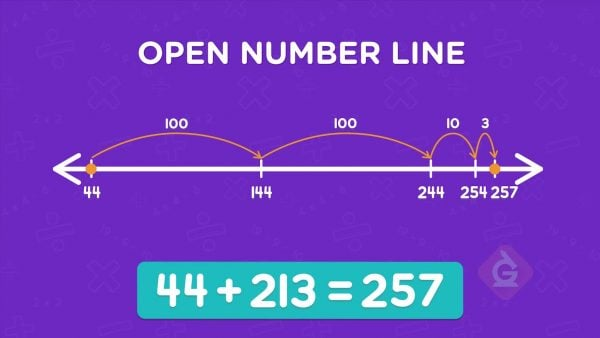A shop sells 213 cookies and 44 cakes. How many items do they sell in all? You can start at either number. One way to add is to make a point at 44 and jump 213. To jump 213 you can jump 100, 100, 10, and 3. You land at 144, 244, 254, and finally 257. 213 + 44 = 257.

Add 3-digit numbers on a number line. A shop sells 213 cookies and 44 cakes. How many items do they sell in all? You can start at either number. One way to add is to make a point at 44 and jump 213. To jump 213 you can jump 100, 100, 10, and 3. You land at 144, 244, 254, and finally 257. 213 + 44 = 257.

### Subtract numbers on a number line.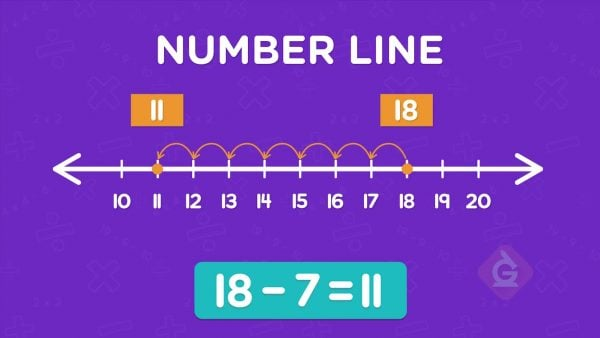You can also subtract numbers on an open number line. To subtract, you place a point at the greater number. Then you jump back to the left, like counting back. To subtract 71 – 32, put a point at 71. Then jump back 32. One way is to jump back 1 to 70. Then 1 more to 69. Then you can jump back 10, 10, and 10 (or 30). The last number you land on is 39. 71 – 32 = 39.

Subtract numbers on a number line. You can also subtract numbers on an open number line. To subtract, you place a point at the greater number. Then you jump back to the left, like counting back. To subtract 71 – 32, put a point at 71. Then jump back 32. One way is to jump back 1 to 70. Then 1 more to 69. Then you can jump back 10, 10, and 10 (or 30). The last number you land on is 39. 71 – 32 = 39.

## INTRO TO THE NUMBER LINE VOCABULARY

Number line
A straight line with numbers placed in increasing order from left to right.
Tick mark
A mark on the number line. Each mark represents a number.
Compare
To determine which number in a set of numbers is greater or greatest.
To combine groups to find a new total.
Subtraction
To take away one number from another.
Open number line
A number line with no tick marks or end points.
Counting by more than 1s (for example, counting by 2s, 5s, 10s, or 100s).

## INTRO TO THE NUMBER LINE DISCUSSION QUESTIONS

### Does a number line always start at 0?

No, a number line can start and end at any number.

### Explain how to add 8 + 7 on a number line with equally-spaced tick marks one unit apart.

I start at the number 8 and make 7 single hops or jumps to the right and land on the number 15.

### Explain how to subtract 290 – 155 on an open number line using skip counting by 100s, 10s, and 5s.

I start at 290 and skip count one hop left of 100 units to land on 190, then I make five hops left of 10 units and land on 140, and then I make one hop left of 5 units to land on 135. 290 – 155 = 135.

### What is one difference between a labeled number line with equal spaces one unit apart and an open number line?

The open number line can show different spacing. I can add very large numbers together on an open number line.

### What is one possible starting point on an open number line that you can use to add 212 + 57?

I can start at 212. I can start at 200 or 210.
X

## Success

We’ve sent you an email with instructions how to reset your password.
Ok